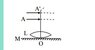# Position of the object and image in this optics problem

PSN03
Homework Statement:
A thin convex lens L (refractive index = 1.5) is placed on a plane mirror M. When a pin is placed at A, such that OA = 18 cm, its real inverted image is formed at A itself, as shown in figure. When a liquid of refractive index μl is put between the lens and the mirror, the pin has to be moved to A', such that OA' = 27 cm, to get its inverted real image at A' itself. The value of μl will be ?
Relevant Equations:
1/f=(μ-1)(1/R-1/R')
I know how to solve the problem but the only thing that's bothering me is what is A?
According to me A is should be 2F ie 2 times the focal length distance. I thought of it like this:
1. First there will be an image formed due to the lens.
2. The image will be formed below the lens but the mirror will reflect the image and the final image will be formed at A again.
3. Since the image formed in the end is inverted and at the same position, hence the distance is 2F.

Am I thinking in the right way? Cause I am not able to get the right answer.

#### Attachments

•Screenshot_2020-08-26_155838.jpg
3.5 KB · Views: 69

Homework Helper
According to me A is should be 2F ie 2 times the focal length distance. I thought of it like this:
1. First there will be an image formed due to the lens.
2. The image will be formed below the lens but the mirror will reflect the image and the final image will be formed at A again.
3. Since the image formed in the end is inverted and at the same position, hence the distance is 2F.

Am I thinking in the right way? Cause I am not able to get the right answer.
If you trace a ray from pin (at 2F) through an edge of the lens, to the mirror and back, where does it go? In particular, what happens on the second pass through the lens?

PSN03
If you trace a ray from pin (at 2F) through an edge of the lens, to the mirror and back, where does it go? In particular, what happens on the second pass through the lens?
Won't it come back to 2F?

Homework Helper
Won't it come back to 2F?
Did you trace it? When the ray leaves the lens on the way down, does it depart at an angle? When it arrives at the lens on the way back up, does it arrive at an angle?

If un-deflected by the lens on the way back up, would the ray hit the pin?
If deflected by the lens on the way back up, would the ray hit the pin?

If ray tracing is not doing it for you, the other way to go is to re-do the algebra taking extreme pains to get the sign conventions right. A virtual image on one side of the lens is not the same as a virtual image on the other.

•PSN03
PSN03
Did you trace it? When the ray leaves the lens on the way down, does it depart at an angle? When it arrives at the lens on the way back up, does it arrive at an angle?

If un-deflected by the lens on the way back up, would the ray hit the pin?
If deflected by the lens on the way back up, would the ray hit the pin?
What I thought was first take just the lens. Let this lens form an image at say B. This B will act as object for the mirror. The reflection of B comes back to A. This was my thinking.

Homework Helper
What I thought was first take just the lens. Let this lens form an image at say B. This B will act as object for the mirror. The reflection of B comes back to A. This was my thinking.
You have three elements in the path: The lens, the mirror and the lens again.

Where is the image after the first pass through the lens?
Where is the image after the reflection? In particular, which side of the lens is it on?
Where is the image after the second pass through the lens, paying careful attention to sign conventions.

•PSN03
PSN03
Did you trace it? When the ray leaves the lens on the way down, does it depart at an angle? When it arrives at the lens on the way back up, does it arrive at an angle?

If un-deflected by the lens on the way back up, would the ray hit the pin?
If deflected by the lens on the way back up, would the ray hit the pin?
I know the point you are telling me is take the rays after passing through lens. These ray will strike the mirror perpendicularly. These perpendicular rays will come back to the lens and hence give the object at same place. But won't this image formed by upright and not inverted? Also what is wrong in my previous way of solving the problem?

Homework Helper
I know the point you are telling me is take the rays after passing through lens. These ray will strike the mirror perpendicularly.
Not if the pin is at 2F they won't.

PSN03
Not if the pin is at 2F they won't.
That will happen when pin is at F